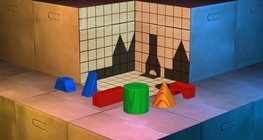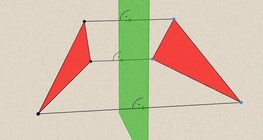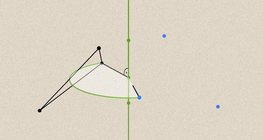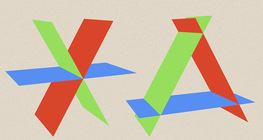Quantity: 0

Total: 0,00

# Geometric transformations – translation

### Geometric transformations – translation

This animation demonstrates geometric translation in both plane and space.

Mathematics

Keywords

translation, geometric transformation, vector, geometric translation in plane, geometric translation in space, geometry, mathematics

### Related itemsThis exciting and colorful game is designed to develop spatial perception. You can check your solutions using isometric views.#### Geometric transformations – reflection

This animation demonstrates geometric reflection, a type of geometric transformation both in plane and space.#### Geometric transformations – rotation

This animation demonstrates geometric rotation, a type of geometric transformation both in plane and space.#### Angle of inclination of lines and planes

This animation demonstrates the angle of inclination of lines and planes in various positions.#### Relative position of lines and planes

This animation demonstrates the relative position of lines and planes.#### Three-dimensional Cartesian coordinate system

3-dimensional Cartesian coordinate system with illustrations and exercises that develop spatial perception.#### Cube

This animation demonstrates the components (vertices, edges, diagonals and faces) of the cube, one of the Platonic solids.#### Dividing Space into Regions by 3 Planes

Division of space by three intersecting planes in several different arrangements.